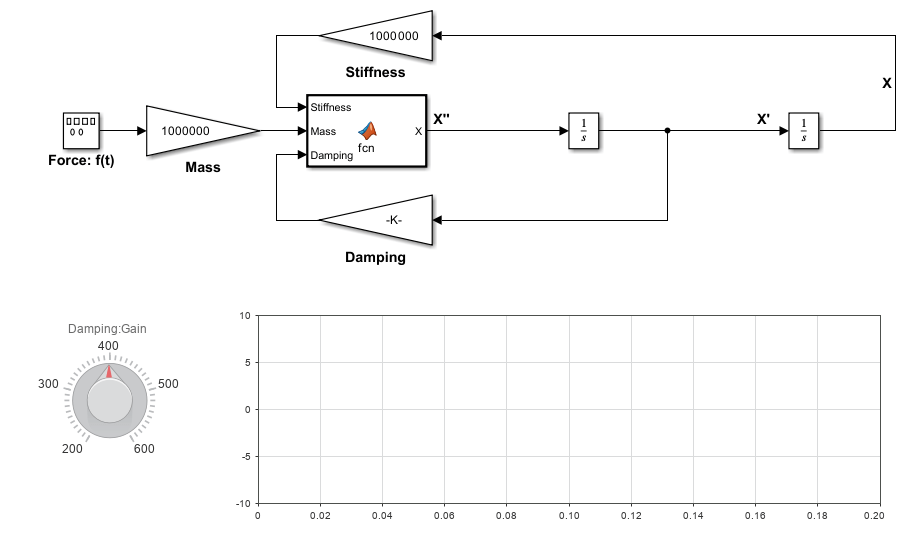# Generate C Code for a Model

To generate C or C++ code from Simulink${}^{®}$ models, Stateflow${}^{®}$ charts, and MATLAB${}^{®}$ functions, use the Simulink Coder™ product. Use the generated code in applications such as simulation acceleration, rapid prototyping, and hardware-in-the-loop (HIL) simulations.

If you are new to Simulink Coder or your application code customization requirements are minimal, you can use graphical tools and default model configuration settings to quickly generate code.

Generating and reviewing code can be as simple as preparing the model for code generation with the Quick Start tool. Then, using code tools accessible from the Simulink Editor, you can configure data interfaces, initiate code generation, and verify the generated code.

This tutorial uses example model `SecondOrderSystem`.

Open the model.

`open_system("SecondOrderSystem");`The model implements a second-order physical system called an ideal mass-spring-damper system. Gain blocks represent components of the system equation: `Mass`, `Stiffness`, and `Damping`. The equation for the system is mX" + cX' + kX = f(t).

• `m` = mass of the system (1.0E-6 kg)

• `c` = damping ratio (4.0e-4 Ns/m)

• `k` = spring stiffness (1.0 N/m)

• `f`(`t`) = forcing function in the x-direction (N)

A Signal Generator block injects a square wave form with an amplitude of 4 and frequency of 20 Hz. The block uses simulation time as the source of values for the waveform time variable. Because the model is configured with a fixed-step solver, which is required for code generation, Simulink uses the same step size for an entire simulation. The consistent step size provides a uniformly sampled representation of the ideal waveform.

The example model shows how you can use MATLAB Function blocks to integrate existing MATLAB function code into Simulink models from which you can generate embeddable C code. The MATLAB function block in the example model integrates a MATLAB function that computes the sum of the component variables.

The Integrator blocks compute integrals of the MATLAB Function block output with respect to time. The solver computes the output of the Integrator block at the current time step, by using the current input value and the value of the state at the previous time step. To support this computational model, the Integrator block saves its output at the current time step for use by the solver to compute its output at the next time step. The block also provides the solver with an initial condition for use in computing the block's initial state at the beginning of a simulation. The default initial condition and the setting for this example model is 0.

The dashboard blocks, Knob and Dashboard Scope, provide visual tooling for tuning the damping and monitoring the waveform. The Knob block is connected to the `Damping` Gain block. The Dashboard Scope block connects to signals `Force: f(t):1` and `X`.

Use this model to learn how to:

1. Generate code by using the Simulink Coder Quick Start tool.

2. Verify whether generated executable program results match simulation results.

3. Tune a parameter during program execution.

4. Deploy prototype code and artifacts.

To start the tutorial, see Generate C Code by Using Simulink Coder Quick Start Tool.